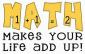# could it be solvable?Help me for the solution of this problem:

What should be the maximum dimensions of a rectangular open box of a given volume V?

• Mathematics inside the configured delimiters is rendered by MathJax. The default math delimiters are $$...$$ and $...$ for displayed mathematics, and $...$ and $...$ for in-line mathematics.